# Linear Regressions and Linear Models using the Iris Data

Have a look at this page where I introduce and plot the Iris data before diving into this topic. To summarise, the data set consists of four measurements (length and width of the petals and sepals) of one hundred and fifty Iris flowers from three species: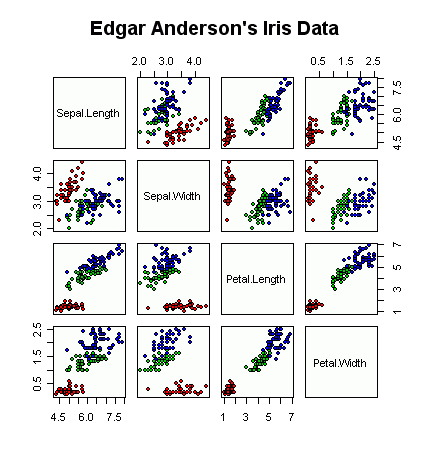## Linear Regressions

You will have noticed on the previous page (or the plot above), that petal length and petal width are highly correlated over all species. How about running a linear regression? First of all, using the "least squares fit" function lsfit gives this:

> lsfit(iris\$Petal.Length, iris\$Petal.Width)\$coefficients
Intercept X
-0.3630755 0.4157554
> plot(iris\$Petal.Length, iris\$Petal.Width, pch=21, bg=c("red","green3","blue")[unclass(iris\$Species)], main="Edgar Anderson's Iris Data", xlab="Petal length", ylab="Petal width")
> abline(lsfit(iris\$Petal.Length, iris\$Petal.Width)\$coefficients, col="black")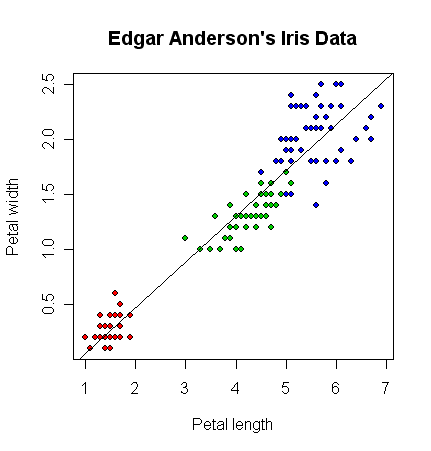The function lsfit is a bit of a "one trick pony" and its a lot more flexible to use a linear model instead (function lm). For this example you get exactly the same thing when we model petal width depending on petal length (written as Petal.Width ~ Petal.Length in R's model syntax):

> lm(Petal.Width ~ Petal.Length, data=iris)\$coefficients
(Intercept) Petal.Length
-0.3630755 0.4157554
> plot(iris\$Petal.Length, iris\$Petal.Width, pch=21, bg=c("red","green3","blue")[unclass(iris\$Species)], main="Edgar Anderson's Iris Data", xlab="Petal length", ylab="Petal width")
> abline(lm(Petal.Width ~ Petal.Length, data=iris)\$coefficients, col="black")

(same graph again)

You get more than just that with a linear model:

```> summary(lm(Petal.Width ~ Petal.Length, data=iris))

Call:
lm(formula = Petal.Width ~ Petal.Length, data = iris)

Residuals:
Min       1Q   Median       3Q      Max
-0.56515 -0.12358 -0.01898  0.13288  0.64272

Coefficients:
Estimate Std. Error t value Pr(>|t|)
(Intercept)  -0.363076   0.039762  -9.131  4.7e-16 ***
Petal.Length  0.415755   0.009582  43.387  < 2e-16 ***
---
Signif. codes:  0 '***' 0.001 '**' 0.01 '*' 0.05 '.' 0.1 ' ' 1

Residual standard error: 0.2065 on 148 degrees of freedom
Multiple R-Squared: 0.9271,     Adjusted R-squared: 0.9266
F-statistic:  1882 on 1 and 148 DF,  p-value: < 2.2e-16 ```

Looking at those p-values, this simple model seems to fit the data very well.

## Linear Models

The main point about using a linear model is we can consider more complicated examples. What about the sepal length as a function of the sepal width?

> plot(iris\$Sepal.Width, iris\$Sepal.Length, pch=21, bg=c("red","green3","blue")[unclass(iris\$Species)], main="Edgar Anderson's Iris Data", xlab="Sepal Width", ylab="Sepal Length")
> abline(lm(Sepal.Length ~ Sepal.Width, data=iris)\$coefficients, col="black")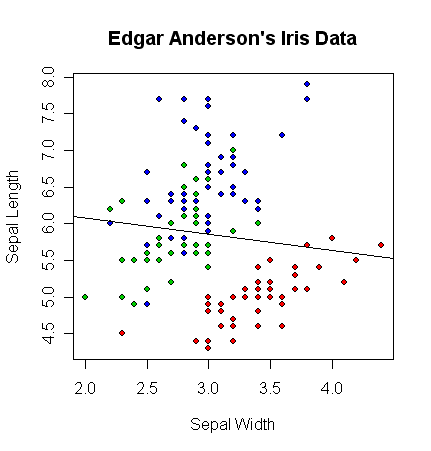It very clear that the linear model Sepal.Length ~ Sepal.Width (black line) is not doing a very good job, even without looking at the statistics:

```> summary(lm(Sepal.Length ~ Sepal.Width, data=iris))

Call:
lm(formula = Sepal.Length ~ Sepal.Width, data = iris)

Residuals:
Min      1Q  Median      3Q     Max
-1.5561 -0.6333 -0.1120  0.5579  2.2225

Coefficients:
Estimate Std. Error t value Pr(>|t|)
(Intercept)   6.5262     0.4789   13.63   <2e-16 ***
Sepal.Width  -0.2234     0.1551   -1.44    0.152
---
Signif. codes:  0 '***' 0.001 '**' 0.01 '*' 0.05 '.' 0.1 ' ' 1

Residual standard error: 0.8251 on 148 degrees of freedom
Multiple R-Squared: 0.01382,    Adjusted R-squared: 0.007159
F-statistic: 2.074 on 1 and 148 DF,  p-value: 0.1519 ```

Look at those lousy p-values.

## Linear Models - Adding terms

What happens if we divide the data up by species, and run three separate linear regressions?

> plot(iris\$Sepal.Width, iris\$Sepal.Length, pch=21, bg=c("red","green3","blue")[unclass(iris\$Species)], main="Edgar Anderson's Iris Data", xlab="Petal length", ylab="Sepal length")
> abline(lm(Sepal.Length ~ Sepal.Width, data=iris)\$coefficients, col="black")
> abline(lm(Sepal.Length ~ Sepal.Width, data=iris[which(iris\$Species=="setosa"),])\$coefficients, col="red")
> abline(lm(Sepal.Length ~ Sepal.Width, data=iris[which(iris\$Species=="versicolor"),])\$coefficients, col="green3")
> abline(lm(Sepal.Length ~ Sepal.Width, data=iris[which(iris\$Species=="virginica"),])\$coefficients, col="blue")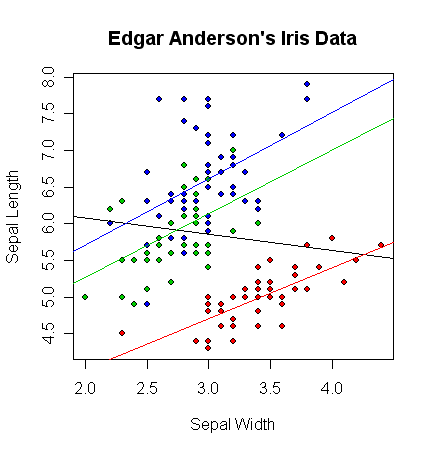The coefficients doing separate per species regressions of Sepal.Length ~ Sepal.Width are:

```> lm(Sepal.Length ~ Sepal.Width, data=iris[which(iris\$Species=="setosa"),])\$coefficients
(Intercept) Sepal.Width
2.6390012   0.6904897
> lm(Sepal.Length ~ Sepal.Width, data=iris[which(iris\$Species=="versicolor"),])\$coefficients
(Intercept) Sepal.Width
3.5397347   0.8650777
> lm(Sepal.Length ~ Sepal.Width, data=iris[which(iris\$Species=="virginica"),])\$coefficients
(Intercept) Sepal.Width
3.9068365   0.9015345```

The equivalent linear model would be something like Sepal.Length ~ Petal.Length:Species + Species - 1, which gives identical coefficients (see later for why I did this):

```> lm(Sepal.Length ~ Sepal.Width:Species + Species - 1, data=iris)\$coefficients
Speciessetosa             Speciesversicolor              Speciesvirginica
2.6390012                     3.5397347                     3.9068365
Sepal.Width:Speciessetosa Sepal.Width:Speciesversicolor  Sepal.Width:Speciesvirginica
0.6904897                     0.8650777                     0.9015345```

What are these new terms? Because Species is a categorical input variable (a factor in R's terminology) it can't be used directly in a linear model as they need actual numbers (a linear model is basically a matrix equation). So, the following "dummy variables" have been invented for each data point (which are just numbers)

• Speciessetosa = 1 if Species is "setosa", 0 otherwise
• Speciesversicolor = 1 if Species is "versicolor", 0 otherwise
• Speciesvirginica = 1 if Species is "virginica", 0 otherwise
• Sepal.Width:Speciessetosa = Sepal.Width if Species is "setosa", 0 otherwise
• Sepal.Width:Speciesversicolor = Sepal.Width if Species is "versicolor", 0 otherwise
• Sepal.Width:Speciesvirginica = Sepal.Width if Species is "virginica", 0 otherwise

Using the summary command on the linear model object gives:

```> summary(lm(Sepal.Length ~ Sepal.Width:Species + Species - 1, data=iris))

Call:
lm(formula = Sepal.Length ~ Sepal.Width:Species + Species - 1,
data = iris)

Residuals:
Min       1Q   Median       3Q      Max
-1.26067 -0.25861 -0.03305  0.18929  1.44917

Coefficients:
Estimate Std. Error t value Pr(>|t|)
Speciessetosa                   2.6390     0.5715   4.618 8.53e-06 ***
Speciesversicolor               3.5397     0.5580   6.343 2.74e-09 ***
Speciesvirginica                3.9068     0.5827   6.705 4.25e-10 ***
Sepal.Width:Speciessetosa       0.6905     0.1657   4.166 5.31e-05 ***
Sepal.Width:Speciesversicolor   0.8651     0.2002   4.321 2.88e-05 ***
Sepal.Width:Speciesvirginica    0.9015     0.1948   4.628 8.16e-06 ***
---
Signif. codes:  0 '***' 0.001 '**' 0.01 '*' 0.05 '.' 0.1 ' ' 1

Residual standard error: 0.4397 on 144 degrees of freedom
Multiple R-Squared: 0.9947,     Adjusted R-squared: 0.9944
F-statistic:  4478 on 6 and 144 DF,  p-value: < 2.2e-16 ```

Just look at those p-values! Every single term has an excellent p-value, as does the model as a whole. And the residual standard error has also been halved.

In this case, the Sepal.Length ~ Sepal.Width:Species + Species - 1 model is clearly much better than just Sepal.Length ~ Sepal.Width.

## Linear Models - Simplify with AIC

On the other hand, what about this choice instead: Sepal.Length ~ Sepal.Width + Species. In fact, this is what the AIC (Akaike Information Criterion) step function gives you if you start with all possible interactions between sepal width and species, which is written Sepal.Length ~ Sepal.Width * Species (using a asterix instead of a plus or colon) in R:

```> summary(step(lm(Sepal.Length ~ Sepal.Width * Species, data=iris)))
...
lm(formula = Sepal.Length ~ Sepal.Width + Species, data = iris)

Residuals:
Min       1Q   Median       3Q      Max
-1.30711 -0.25713 -0.05325  0.19542  1.41253

Coefficients:
Estimate Std. Error t value Pr(>|t|)
(Intercept)         2.2514     0.3698   6.089 9.57e-09 ***
Sepal.Width         0.8036     0.1063   7.557 4.19e-12 ***
Speciesversicolor   1.4587     0.1121  13.012  < 2e-16 ***
Speciesvirginica    1.9468     0.1000  19.465  < 2e-16 ***
---
Signif. codes:  0 '***' 0.001 '**' 0.01 '*' 0.05 '.' 0.1 ' ' 1

Residual standard error: 0.438 on 146 degrees of freedom
Multiple R-Squared: 0.7259,     Adjusted R-squared: 0.7203
F-statistic: 128.9 on 3 and 146 DF,  p-value: < 2.2e-16 ```

How can we interpret this? Recall we did three species specific regressions? If you imagine applying a shift of 1.4587 to the Iris versicolor (green) points, and a shift of 1.9468 to the Iris virginica (blue) points then their individual best fit lines would more of less agree (with a gradient of say 0.8036):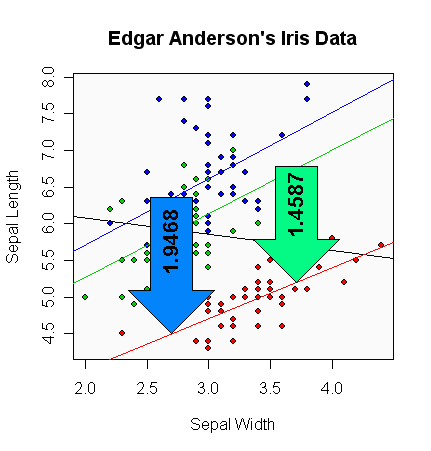Or, to put that another way, this model predicts that Sepal.Length = (0.8036 * Sepal.Width) + 2.2514 plus an additional 1.4587 if the species is Iris versicolor or 1.9468 if the species is Iris virginica.

Note that using summary(step(lm(Sepal.Length ~ Sepal.Width * Species - 1, data=iris))) gives an equivalent model, but like the case discussed below would use a dummy variable for each of the three species, rather than an intercept term and two dummy variables.

## Note on Intercept Coefficients

Recall that I just introduced a model of the form Sepal.Length ~ Sepal.Width:Species + Species - 1, which gave identical coefficients to those found doing species specific regressions:

```> lm(Sepal.Length ~ Sepal.Width:Species + Species - 1, data=iris)\$coefficients
Speciessetosa             Speciesversicolor              Speciesvirginica
2.6390012                     3.5397347                     3.9068365
Sepal.Width:Speciessetosa Sepal.Width:Speciesversicolor  Sepal.Width:Speciesvirginica
0.6904897                     0.8650777                     0.9015345
```

The use of the "- 1" in the model above told R not to automatically include a default intercept term. The alternative is the following:

```> lm(Sepal.Length ~ Sepal.Width:Species + Species, data=iris)\$coefficients
(Intercept)             Speciesversicolor              Speciesvirginica
2.6390012                     0.9007335                     1.2678352
Sepal.Width:Speciessetosa Sepal.Width:Speciesversicolor  Sepal.Width:Speciesvirginica
0.6904897                     0.8650777                     0.9015345```

This time the dummy variables are:

• (Intercept) = 1, regardless of Species
• Speciesversicolor = 1 if Species is "versicolor", 0 otherwise
• Speciesvirginica = 1 if Species is "virginica", 0 otherwise
• Sepal.Width:Speciessetosa as before
• Sepal.Width:Speciesversicolor as before
• Sepal.Width:Speciesvirginica as before

Notice that the new (Intercept) term and the old Speciessetosa have the same coefficient, 2.6390012. Similarly note that for Speciesversicolor the old value of 3.5397347 equals 2.6390012 + 0.9007335 (the intercept plus the new coefficient), and likewise for Speciesvirginica, the old value of 3.9068365 equals 2.6390012 + 1.2678352. This all makes sense - these are basically equivalent models but with different dummy variables. Anyway, I found the first case slightly easier to explain.